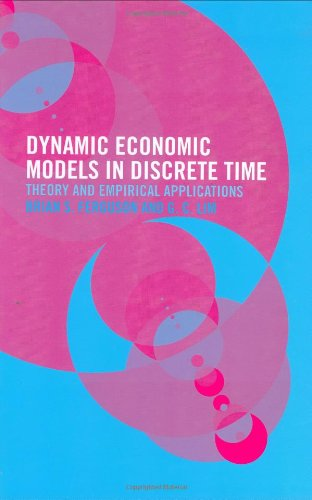# The Strange Logic of Random Graphs

Warning: mysql_num_rows() expects parameter 1 to be resource, boolean given in /home/web/naiti.in/lib.php on line 113Автор Joel Spencer
 Год 2001
 Страниц 90
 ISBN ISBN10:3540416544
 Издатель Springer### Описание:

The study of random graphs was begun by Paul Erdos and Alfred Renyi in the 1960s and now has a comprehensive literature. A compelling element has been the threshold function, a short range in which events rapidly move from almost certainly false to almost certainly true. This book now joins the study of random graphs (and other random discrete objects) with mathematical logic. The possible threshold phenomena are studied for all statements expressible in a given language. Often there is a zero-one law, that every statement holds with probability near zero or near one. The methodologies involve probability, discrete structures and logic, with an emphasis on discrete structures. The book will be of interest to graduate students and researchers in discrete mathematics.

### Похожие книгиThe strange logic of random graphsАвтор: Joel SpencerГод: 2001Introduction to Sequential Dynamical SystemsАвтор: Henning S. MortveitГод: 2007Dynamic Graph AlgorithmsАвтор: Eppstein D., Galil Z., Italiano F.Dynamic economic models in discrete timeАвтор: Ferguson B.S., Lim G.C.Год: 2003Computational discrete mathematics: advanced lecturesАвтор: Alt HelmutГод: 2001Computational discrete mathematics. Advanced lecturesАвтор: Alt H. (ed.)Год: 2001